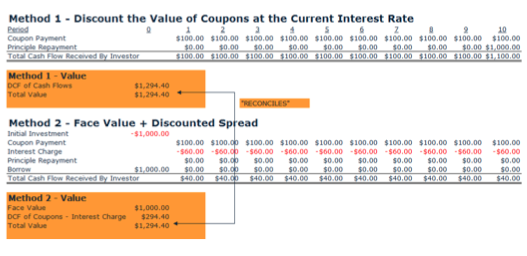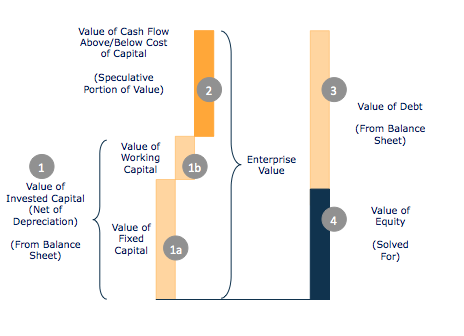Theoretically, stocks trade at the present value of future cash flows accruing to shareholders. However, future cash flows cannot be forecast with certainty. If investors knew with certainty what future cash flows would be, they would pay exactly the same price for a stock. A similar example of this is a bond. The value of a bond is easy to calculate given the coupon rate, the prevailing interest rate and the face value (i.e. salvage value). To calculate the value of the bond, all coupons payments and the face value are discounted to the present value using the prevailing interest rate to derive the price of the bond that every rational investor would pay.

A bond market only exists because of credit risk and fluctuating interest rates (variables that cannot be predicted with certainty). In the absence of credit risk and fluctuating interest rates, we wouldn’t have a bond market because everyone would know the true economic value rendering a market unnecessary.
Stocks are no different than bonds, except that future cash flows for stocks have a greater degree of uncertainty.
Consider a bond with the following variables:

A) Face Value = \$1,000
B) Life = 10 years
C) Coupon Rate = 10%
D) Annual Coupon = \$100
E) Market Interest Rate = 6%

This bond can be valued in two ways:Most people are familiar with Method 1 because it is straightforward. Most people are also surprised that Method 2 provides the same answer. More importantly, Method 2 is more insightful because it shows “value added” over time. We show Method 2 because it is the basis for how we value stocks.
Many of the same principles apply in valuing a stock as in valuing a bond. The Face Value of the bond is similar to the Net Invested Capital in the company. The Coupon of the bond is similar to the Cash Flow from Operations in the company. And, the Interest Charge is similar to a Capital Charge in the company. Stocks are more complicated because of growth and uncertainty that we will discuss later.

### VALUATION BREAKDOWN

The figure below shows the breakdown of our methodology in valuing a company.### 1) Value of Inflation Adjusted Net Invested Capital

Recall that in performance measurement GROSS invested capital was the appropriate variable to use in calculating CFIRR. However in valuation, it is appropriate to use NET invested capital. At this point we want to know how much the company is worth. If assets are old and depreciated we need to take this into account. The line items included here are the same as performance measurement for inflation adjusted gross capital with the exception that it is after accumulated depreciation. It is worth noting that the methodology separates out inflation adjusted net invested capital into 1a) working capital and 1b) fixed capital.

### 2) Forecast – Value of Cash Flow Above/Below the Cost of Capital (SEE FORECASTING SECTION)

This could also be called the “value added” and is the speculative portion of value.
Forecasts of CFIRR and capital growth will create value or destroy value. If a forecast is a positive spread (i.e. CFIRR – Cost of Capital is positive), then value is created. In this case, the higher the capital growth forecast, the higher the valuation creation. If a forecast is a negative spread (i.e. CFIRR – Cost of Capital is negative) then value is destroyed. In this case, the higher the capital growth forecast, the higher the value destruction. If a forecast is a zero spread (i.e. CFIRR – Cost of Capital is zero), then growth does not affect value.

### 3) Value of Debt

The value of debt (more accurately value of debt and equivalents) is calculated from the balance sheet as follows:
Value of Debt =
+ Long-Term Debt
+ Minority Interest (from Balance Sheet)
+ Other Non-Current Liabilities (including Pension liabilities)
+ Preferred Stock
+ Short-Term Debt
Other items that could be added to value of debt would be off balance sheet liabilities such as pending lawsuits and under-funded pensions.

### 4) Value of Equity

All the above calculations lead to the value of the equity. The CFIRR and capital growth history lead to the CFIRR and capital growth forecasts, including the fade. The forecast produces a stream of cash flows less the cost of capital that is discounted to the present value. These discounted cash flows are added to the value of net invested capital to produce the total enterprise value. Finally, the value of equity is calculated as the total enterprise value less the value of debt.

### Cost of Capital

This is the most contentious issue in finance and in our methodology. Our cost of capital may seem simple (which is one of its advantages), but there are very good reasons for the methodology, which uses the 30-year Treasury bond rate. The cost of capital, or discount rate, is used for two primary purposes: 1) to account for the time value of money; and, 2) to account for risk.
It is fair to say the time value of money is the same regardless of what company you are valuing. The risk of each company varies, but it is better to model risk in the cash flows rather than in the cost of capital. To do this, we apply unique fade rates and conservative cash flow estimates for each company (as discussed) in order to perform rigorous scenario analyses to account for risk. This makes comparisons from company to company much easier. In debating the value of companies, we would prefer to discuss fade rates and scenarios rather than the appropriate discount rate.
Sometimes the cost of equity can be lower than the cost of debt. Companies typically raise equity when their stock price is high, resulting in minimal dilution for existing shareholders. Companies raising capital can assess the cost of debt versus the cost of equity by comparing interest rate cost to dilution cost. Furthermore, when companies are losing money, raising equity will dilute a loss (i.e. more shareholders sharing the pain). On average, companies will raise money at or near the 30-year Treasury bond rate.